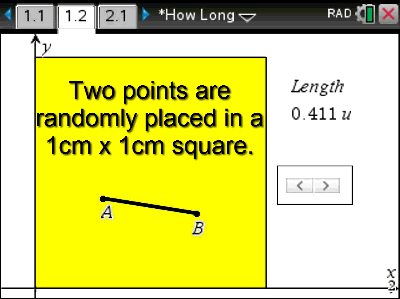# Activities

•• ##### Subject Area

• Standard: National Curriculum 7-10: Number and Algebra: Linear and non-Linear Relationships

• ##### AuthorMiddle

60 Minutes

• ##### Device
•TI-Nspire™ CX
•TI-Nspire™ CX CAS
• TI-Nspire™ Apps for iPad®
• ##### Software

TI-Nspire™
TI-Nspire™ CAS

4.3

## How Long#### Activity Overview

Two points are placed randomly in a 1cm x 1cm square. What would be the average distance between these two points? This wonderful problem has been constructed as an applicable exploration that uses Pythagoras’s theorem; however, extension opportunities reach well beyond year 12 mathematics. So what’s your guess? Fancy exploring the problem in 3D?

#### Objectives

• ACMNA214 – Find the distance between two points located on a Cartesian plane using a range of strategies, including graphing software.
• ACMMG276 - Pythagoras' theorem and trigonometry to solving three dimensional problems in right-angled triangles (Extension Option)

• Pythagoras
• Random
• Mean

#### About the Lesson

Students start by estimating the answer to the problem: Two points are placed randomly inside a 1cm x 1cm square, what would be the average length of the line joining these two points. Following their estimation they use the Cartesian plane to plot points randomly and derive the equation for the distance between two points. Students then use the spreadsheet application to build a much bigger sampling tool. Upon completion students are then able to explore bigger squares, rectangles or tackle the extension option exploring the length of a similar line placed in a 3D box.